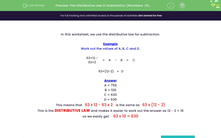# The Distributive Law in Subtraction (Numbers <100)

In this worksheet, students use the distributive law to answer subtraction questions.Key stage:  KS 3

Curriculum topic:   Number

Curriculum subtopic:   Use Relationships Between Operations

Difficulty level:#### Worksheet Overview

In this worksheet, we use the distributive law for subtraction.

Example

Work out the values of A, B, C and D.

63×12 - 63×2 =
 A
-
 B
=
 C

63×(12-2) =
 D

A = 756
B = 126
C = 630
D = 630

This means that   63 x 12 - 63 x 2   is the same as   63 x (12 - 2)

This is the DISTRIBUTIVE LAW and makes it easier to work out the answer as 12 - 2 = 10

so we easily get     63 x 10 = 630

### What is EdPlace?

We're your National Curriculum aligned online education content provider helping each child succeed in English, maths and science from year 1 to GCSE. With an EdPlace account you’ll be able to track and measure progress, helping each child achieve their best. We build confidence and attainment by personalising each child’s learning at a level that suits them.

Get started